# Top 8 Best Algebra 2 Courses (Online)by Hannah Beams | Last Updated:   November 29, 2021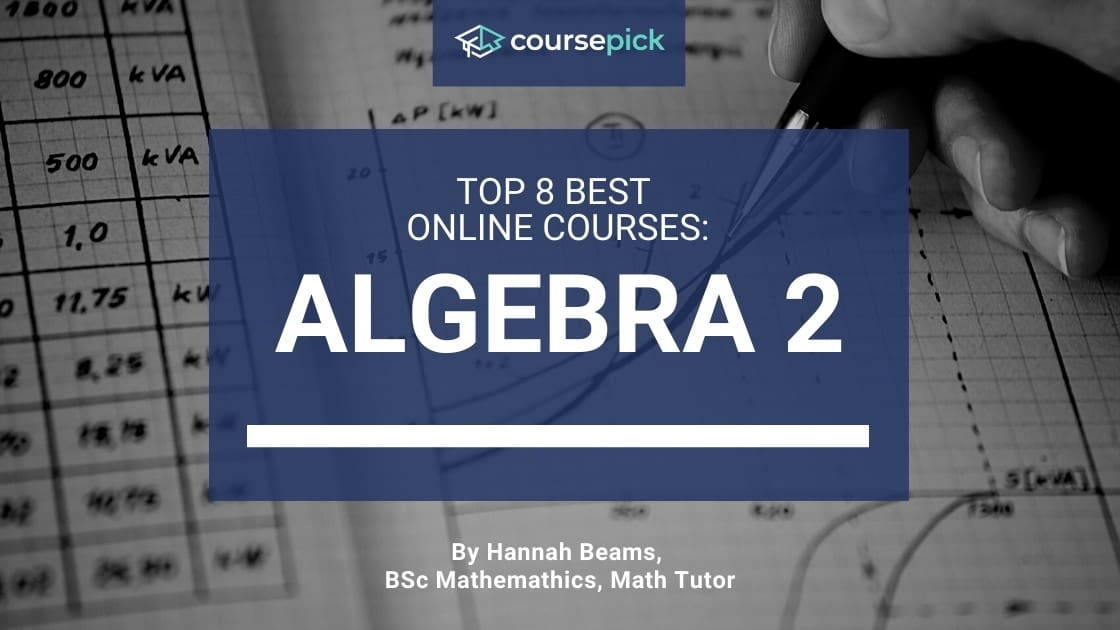There are many resources available online that a student can use to study Algebra 2, for this article, I selected courses containing a varying number of lectures, guided examples, practice problems, and practice tests.

It is best to keep in mind the learning style of the student when selecting a course. Students who are visual and/or aural learners will benefit the most from online courses that offer a large number of lecture videos.

Students who are verbal learners may benefit from a course that offers reading material or taking a course that is supplemented by a textbook.

Students who are more physical learners may benefit from courses that offer a large number of practice problems that challenge the student to work through the material on paper.

## Become an Algebra Master – Krista KingThe course Become an Algebra Master is a course that covers both Algebra 1 and Algebra 2.

It is available on Udemy and is taught by Krista King. Krista has structured the course to make the complicated concepts of Algebra 2 accessible to students who have a large range of problem solving backgrounds.

A student taking this course has access to all 125 quizzes, 21 workbooks, and over 14 hours of lectures that explain the concepts within both Algebra 1 and Algebra 2.

The Algebra 2 portion of this course is broken into 11 units and a final exam.

The units cover exponents and radicals, ratio and proportion, complex fractions, rational functions, imaginary numbers, advanced equations, systems of equations, factoring, graphing, manipulating functions, and exponential and logarithmic functions.

All of the materials provided by Krista gives the student a variety of ways to study. This allows students who have all different forms of learning styles to benefit from taking this course.

A student will learn the most from this course if they already have an understanding of how to use arithmetic operations on whole numbers, decimals, fractions, exponents, and radicals.

With access to over 1300 practice problems, students taking this course will have ample material to learn Algebra 2.

The lectures within this course present the major concepts on a virtual whiteboard with accompanying audio that helps explain the illustrated material.

These lectures are then coupled with quizzes and step-by-step walkthroughs of solutions. The structure of this course fits most learning styles.

Krista’s in depth detailed explanations and large amounts of practice work available make this a class best for a student looking to really dive into each concept of Algebra 2.

Upon completion of Become an Algebra Master students will receive a certificate of completion from Udemy.

## Algebra 2: The Complete Course – Kashif A.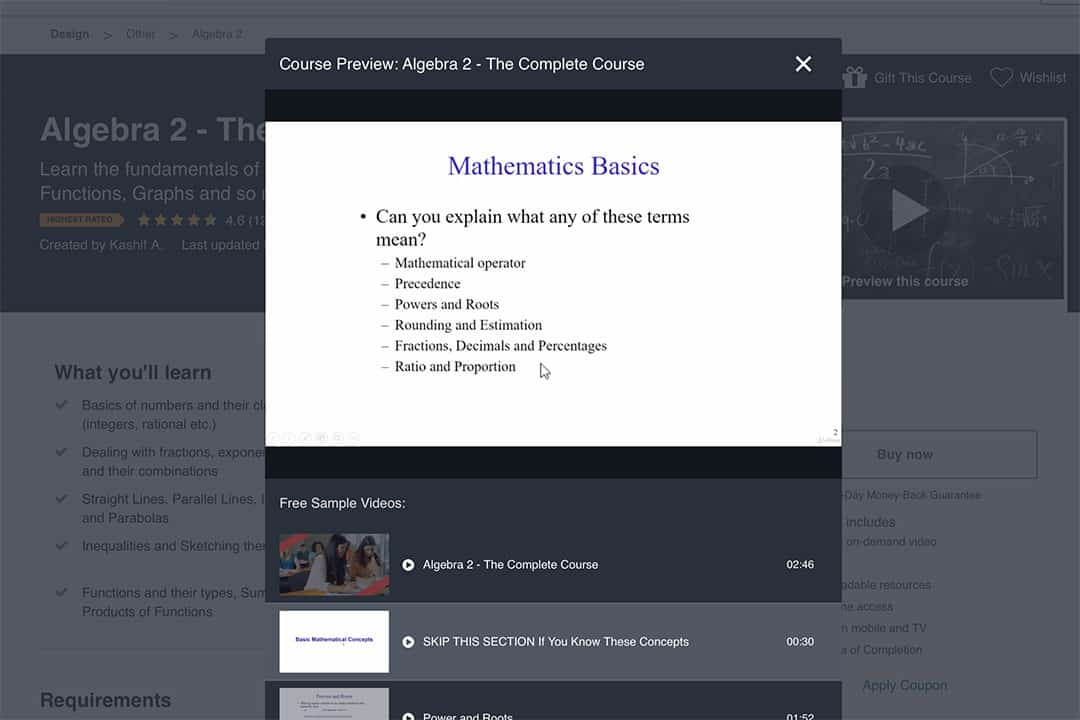The instructor, Kashif A., has taught for 11 years at a university level and holds a master’s degree in Engineering.

Kashif structures the course to be broken into 76 lectures that cover three main units: Basic Mathematical Concepts, Coordinate Geometry and Straight Lines, and Functions, Graphs, and Transformations.

The course material is available in the form of lectures that offer examples, activities, and summaries of the material covered.

The 4 and a half hours of lecture available to students in this course go over arithmetic and the order of operations; like terms; exponents, indices, and surds; types of functions and their graphs; inequalities and their graphs; solving systems of equations; and polynomials and factorization.

If the student is already familiar with all sections in the Basic Mathematical Concepts unit, Kashif recommends that the student begins the course with unit Coordinate Geometry and Straight Lines.

Students who choose to take this course will be able to learn as well as practice all of the concepts typically taught within Algebra 2.

The lectures, explained examples, and activities available in this course makes this course most suitable to students who are visual and aural learners.

These lessons are structured as power point videos that have clear, concise descriptions of the course material. Students who are already familiar with arithmetic, fractions, and exponents will benefit the most from this course.

Once the student has finished Algebra 2—The Complete Course the student will receive a certificate of completion from Udemy.

## Algebra 2 & Trigonometry: A Complete High School Curriculum – Matthew Gromlich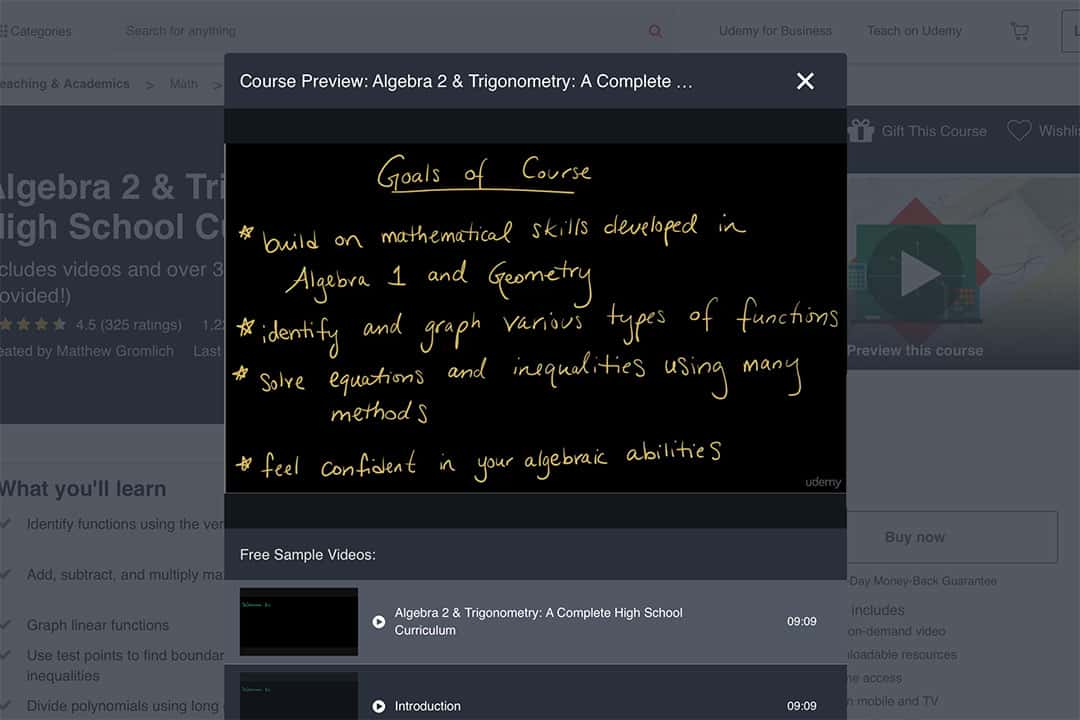The course Algebra 2 & Trigonometry: A Complete High School Curriculum covers all Algebra 2 concepts.

Towards the end of the course some trigonometry concepts are also incorporated. This course is also available on Udemy and is taught by Matthew Gromlich.

Matthew has 4 years of teaching experience and is currently a Ph.D. candidate in Curriculum and Instruction with an emphasis in Math Education at the University of Nevada.

His course covers 17 core units that in total have 16 hours of lectures and over 300 pages of quizzes and worksheets.

The units cover topics such as matrices and their properties, absolute values and inequalities, solving for and working with linear and quadratic functions, systems of equations and inequalities, trigonometric functions, the unit circle and properties of angles, and combinatorics.

Once a student has worked through each of the units in this course, they will have covered all of the material typically covered in a high school Algebra 2 class.

This course is designed to have students watch the video lectures provided and then practice the skills they are learning with the provided worksheets.

The lectures provided are audio explanations of work written visually on a virtual white board. This allows for students who are verbal learners to benefit from reading the work.

Students who are aural learners will enjoy the audio associated with each video.

Students who wish to have a more kinesthetic learning approach can take notes from each video.

The worksheets and quizzes also allow for more physical, on-paper practice that students can take advantage of.

Students who have already taken Algebra 1 and Geometry will benefit the most from this course. Upon completion of this course the student will receive a certificate of completion from Udemy.

## Algebra II Explained – John Greene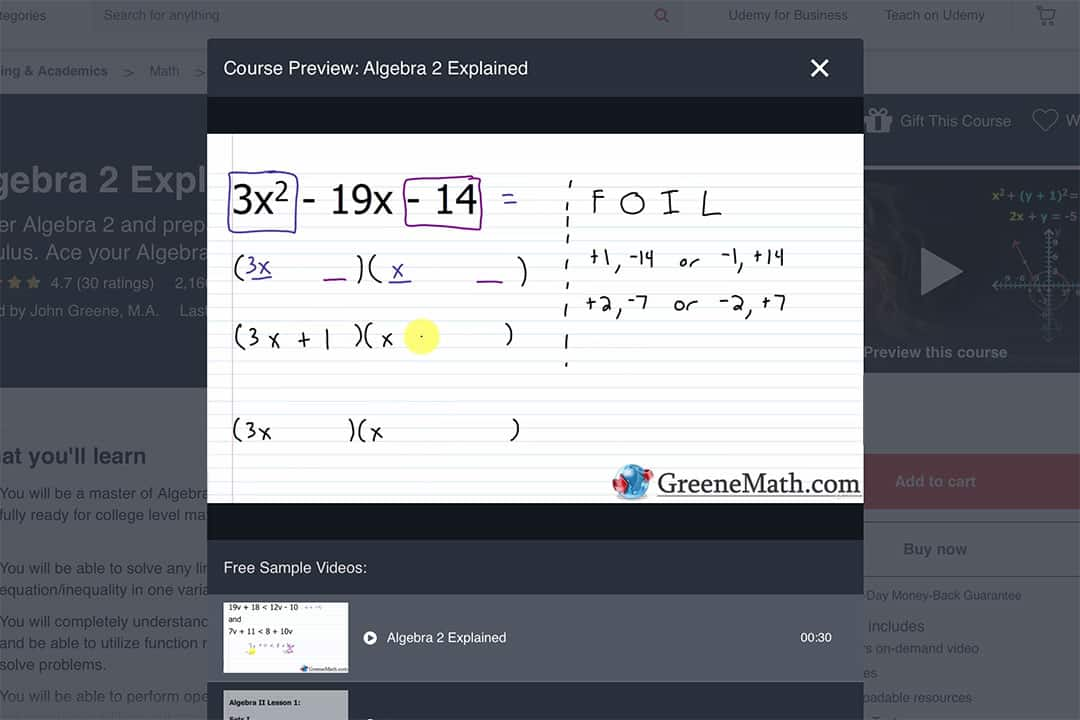The course Algebra 2 Explained covers all concepts typically taught within a high school Algebra 2 course. It is designed for students who are preparing for Trigonometry, Pre-Calculus, and Calculus.

This course is available on Udemy and is taught by John Greene. John has a bachelor’s degree in Mathematics as well as a master’s degree in Economics.

John’s course has 15 units that each have lectures, practice questions, study notes, and practice tests. The units in John’s course cover sets, working with and graphing linear equations and inequalities, systems of equations and inequalities, quadratic equations and polynomials, factoring, functions, inverse functions, exponential and logarithmic functions, variation, rational expressions, and radicals.

Students taking this course have access to 240 lectures that contain over 40 hours of content.

Students interested in taking this course should be comfortable with Pre-Algebra was well as basic arithmetic. John’s lectures are a combination of PowerPoint slides that highlight key information, and virtual whiteboard illustrations of example problems.

The detail-oriented lectures as well as the 1000 practice problems available to students make this course a good option for students looking to learn Algebra 2.

The varied lecture styles, and access to ample practice problems will give students of all learning styles clarity on each challenging topic. Once the course has been completed, students will receive a certificate of completion from Udemy.

## The Complete Guide to Algebra 2 – Mile O-HalloranThe course The Complete Guide to Algebra 2 is available for any students wishing to practice their problem solving and Algebra 2 skills.

Mike O’Halloran teaches this course, and students have access to 12 units of material that contain over 28 hours of lecture.

Mike is a high school math teacher and SAT/ACT math tutor who hopes to make math more enjoyable and rewarding for each of his students.

Each unit contains lectures, practice problems, and tests that are designed to challenge the student in their learning process.

The units cover solving equations, solving systems of equations and matrices, quadratic equations and polynomials, functions, exponential and logarithmic functions, radicals and roots, rational expressions, conic sections, and sequences and series.

Students already comfortable with Pre-algebra and Algebra 1 are best prepared to take this course. Mike has created the lectures for this course as power point slides that he annotates and discusses in each video.

His step by step explanations of concepts and attention to the problem solving process make this course a great option for students looking to improve their problem solving skills.

Taking this course will give students the resources to master Algebra 2, help prepare for the SAT and ACT, and develop a problem-solving mindset.

Upon completion of this course student will receive a certificate of completion from Udemy.

## Algebra II (Intermediate Algebra) – Math Fortress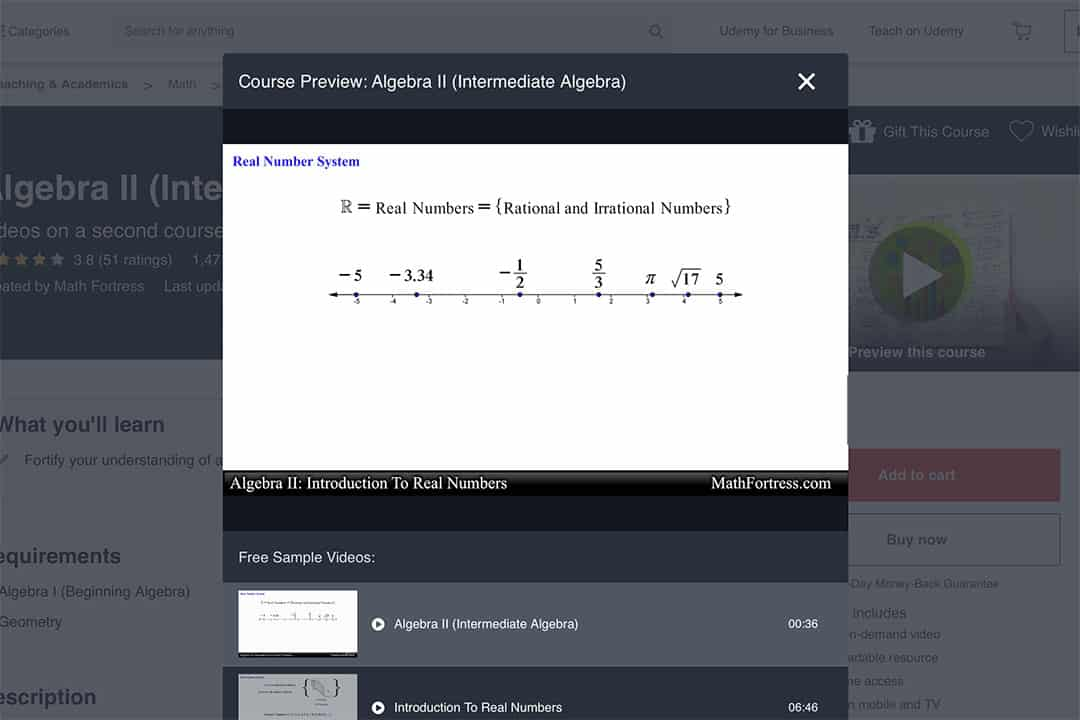The course Algebra II (Intermediate Algebra) is available on Udemy for students looking to strengthen their understanding of algebra 2 and practice their problem solving skills.

This course is designed for high school and college students who already have an understanding of Algebra 1 and Geometry.

The material covered by this course is intended to either stand alone as a resource for students learning Algebra 2 or supplement the student’s current Algebra 2 class.

The course covers an introduction to real numbers and linear equations, and quadratic equations in about 2 hours of video that is broken into 16 lectures.

The lectures within this course are PowerPoint slides accompanied by verbal explanations. The slides in each lecture present vocabulary, definitions, and a multitude of examples that are discussed in step-by-step explanations.

This course best suits students who learn through verbal and aural lessons. Once a student finishes this course, they will receive a certificate of completion from Udemy.

## Algebra 2 Video Series – Jesse PatrickThe course Algebra 2 Video Series is available for students on Udemy.

Unlike some of the courses available on Udemy, this course is a collection of videos rather than a structured setup with practice problems and tests.

This course has been designed by Jesse Patrick who is an experienced high school math teacher and SAT math tutor. Jesse’s videos cover 10 units of Algebra 2 material.

The 10 units in this course cover equations and inequalities, linear functions and systems of equations, parent functions and transformations, quadratic equations and complex solutions, polynomial functions, radical functions, exponential and logarithmic functions, rational functions, conic sections, sequences and series, probability and statistics, and trigonometry.

Students interested in taking this course should already be comfortable with Algebra 1. With access to 80 video lectures that in total go over about 34 hours of material, this course is best suited for students who have a visual/verbal learning style.

The lectures available within the course are PowerPoint slides that Jesse annotates and discusses in accompanying audio.

Students who learn the most from listening to aurally presented information will be very well supported by this course. Upon completion of this course students will receive a certificate of completion from Udemy.

## Learn Algebra The Easy Way! – Julio Gonzales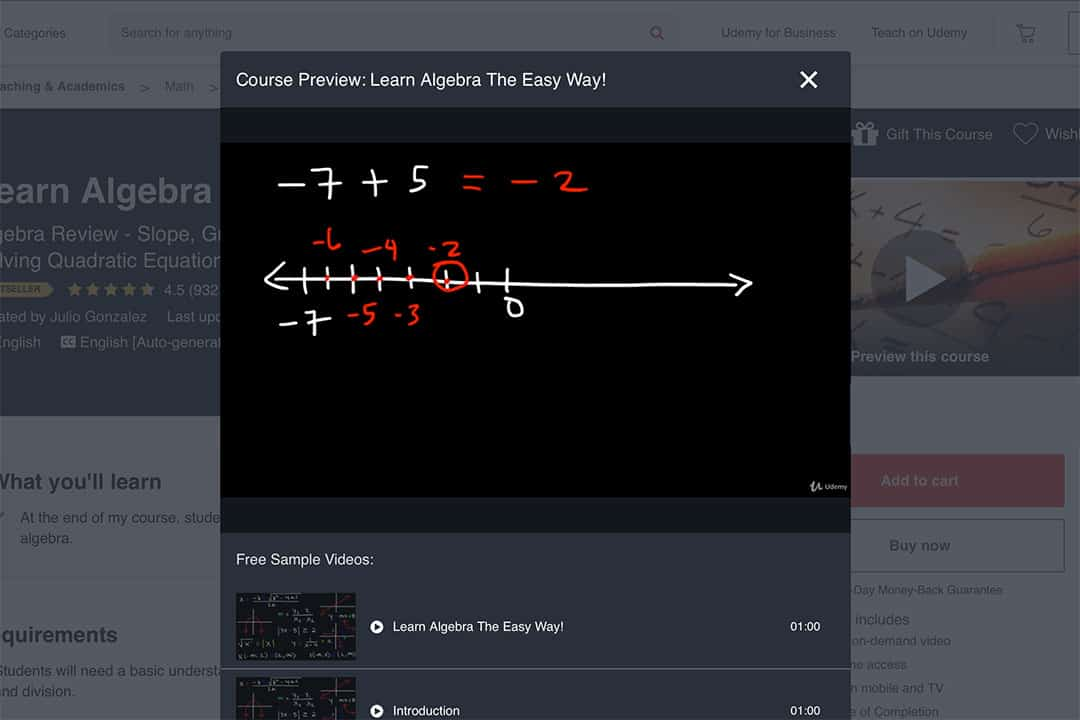The course Learn Algebra The Easy Way! can be accessed by students on Udemy. This course is taught by Julio Gonzalez. Julio has a BA in chemistry and has spent many years tutoring problem solving heavy topics including Algebra 2.

Julio’s course has 18 units that are broken down into 227 lectures which cover over 20 hours of material. Students that choose to take this course have access to material that will help them develop the skills needed for both Algebra 1 and Algebra 2.

The units available to students in this course that cover Algebra 1 focus on basic arithmetic, fraction rules, and operations.

The units that focus on Algebra 2 delve into solving and graphing linear equations, systems of linear equations, linear equalities and absolute value expressions, polynomials, factoring, quadratic equations, rational expressions, radical expressions, complex imaginary numbers, exponential and logarithmic functions, functions, and conic sections, and arithmetic and geometric sequences.

Students who wish to take this course should already be comfortable with basic arithmetic. Julio’s lectures are whiteboard illustrations of material and problems.

Students who are aural and/or physical learners will enjoy the detailed audio explanations and the ability to take detailed notes from the lectures. Once the student completes this course, they will receive a certificate of completion from Udemy.

## How to pick the right Algebra 2 course?

Students who are interested in studying Algebra 2 should consider what they are trying to get out of their learning. Is the student looking to strengthen their problem solving skills?

Is the student interested in taking Algebra 2 so that they can prepare for standardized tests such as the SAT and the ACT?

Is the student interested in taking Algebra 2 so that they can be prepared for courses like Trigonometry, Pre-Calculus, or Calculus?

Students looking to strengthen their problem solving skills should take an online course that provides a variety of practice problems.

Taking the time to work through problems and examples within the course will give the student the chance to develop the core foundation of skills that are required for algebra problems.

When it comes to math problems, repetition is one of the best study tools. Working through many practice problems that are attached to a given lesson will help the student practice the concepts from a variety of contexts.

Students studying Algebra 2 to prepare for standardized tests should be sure to take a course online that provides in-depth explanations as well as ample practice.

Standardized tests such as the SAT and ACT are used to measure the student’s current knowledge of math, science, reading, and writing. Students who are preparing for the math section of these tests will benefit the most from courses that are geared towards improving a student’s ability to solve Algebra 2 problems.

Students looking to study Algebra 2 as a means of preparing for upper-level math courses should look at courses that best fit their learning style.

A student will learn better in an environment that suits their most productive mode of learning. Algebra 2 is a foundational course that is necessary for most advanced math classes. The topics taught in Algebra 2 are foundational concepts that the student will use time and time again in their later course work.

Another good source of information and practice when studying algebra are books like Algebra II For Dummies. This book explains Algebra 2 concepts in lessons that break down each concept into easy to follow components.

The book also provides step-by-step explanations for example problems found within each lesson that students can complete as they work through each lesson.

### What is Algebra 2?

Algebra is a tool that helps recognize patterns and find solutions to real-life problems.

Taking the time to practice the concepts needed to solve algebra problems gives a student the ability to think critically about advanced problem solving concepts.

Algebra 1 focuses on solving equations and Algebra 2 continues this focus while adding additional complexity. This area of math focuses on topics like linear equations, quadratic equations, inequalities, absolute values, logarithms, radical functions, sequences, series, and more. The broad scope of this class comes from Algebra’s various applications. Mastering Algebra 2 provides students with a mathematical toolbelt that will help them with their future problem solving work.

A student learns how to solve many different types of equations while studying Algebra 2.

As previously stated, the types of equations that are covered in Algebra 2 include linear equations, quadratic equations, and functions, and these equations can be used to model the mathematical relationships between variables.

For example, linear equations can be used to convert the temperature from Fahrenheit to Celsius; a quadratic equation can predict the arc that a baseball takes when it is thrown in the air, and an employee’s weekly pay is a function of that employee’s hourly rate and the number of hours that they worked.

### Is Algebra 2 an important subject to know?

Algebra 2 is a foundational course for all students. It teaches problem solving skills that will become integral for the student’s learning process and growth in their education.

Robert Talbert discusses the importance of Algebra 2 in his article What to do about Algebra 2.

Robert, a math professor who is passionate about teaching practices, acknowledges how educational systems do not agree on whether Algebra 2 should be considered a core course.

His article discusses the importance of problem solving, understanding, and mathematic training. Robert states that, “If you get rid of Algebra 2 to remove the burden of symbolic manipulation skill, you also get rid of the above ideas, unless you create something else in its place”.

### Why do Algebra 2 concepts have such a wide range?

Algebra 2 covers a wide variety of concepts so that students are familiar with different situations that require this level of problem solving.

The information detailed in Algebra II Chapter of the Mathematics Framework for California Public Schools: Kindergarten through Grade Twelve states how in an Algebra 2 class “students work closely with the expressions that define the functions and continue to expand and hone their abilities to model situations and to solve equations”.

Working through the range of concepts taught in Algebra 2 prepares a student to learn the framework of problem solving math that extends to areas such as Calculus, Probability and Statistics, Linear Algebra, and more.

### How does Algebra 2 compare with Trigonometry and Pre-Calculus?

Algebra 2 is typically a prerequisite for Trigonometry and Pre-Calculus courses.

Trigonometry builds upon the concepts of solving equations and inequalities and applies them to the study of angles and triangles.

Pre-Calculus is a course that prepares a student for Calculus coursework by covering and expanding upon Algebra 2, Trigonometry, and Geometry concepts.

## Conclusion

Studying Algebra 2 gives a student hands on experience with problem solving.

The courses listed in this article provide students with a variety of methods of learning and practicing this material.

As long as a student is familiar with basic arithmetic, there are courses available that will help provide the student with the right resources to study this area of math.

Algebra 2 is a foundational course that prepares students for further math work in upper level courses, college entrance exams, and problem solving skills as a whole.

`